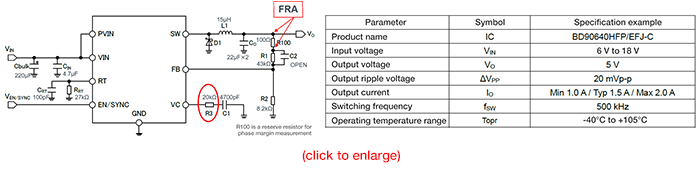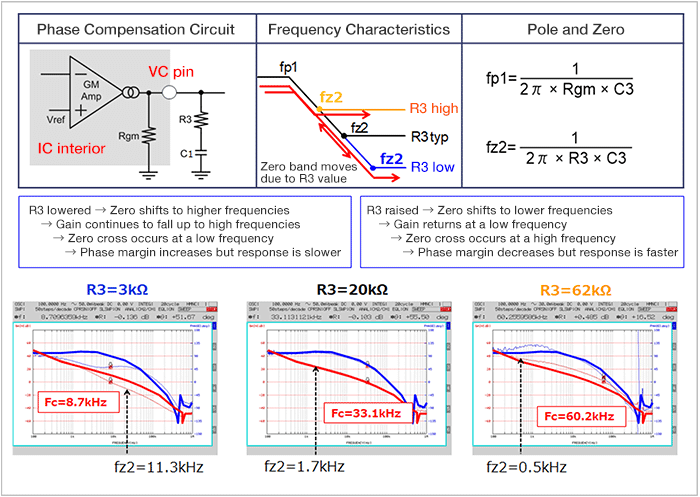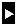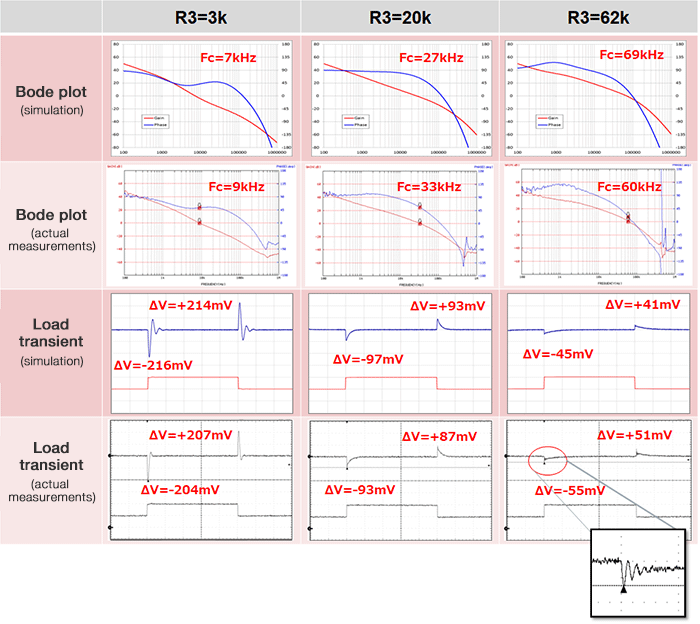Technical Information Site of Power Supply Design

• TECH INFO
• Optimization of Frequency Characteristics of a DC/DC Converter in the Design Stage : Method of Setting Phase Compensation and Use of Simulations -Part 2-

Optimization of Frequency Characteristics of a DC/DC Converter in the Design Stage

# Method of Setting Phase Compensation and Use of Simulations

## -Part 2-

Keyword Simulator available free of chargeBD90640EFJVC pinPhase compensation resistor Phase compensation capacitorFeedback loop phase compensationFrequency characteristic adjustmentPhase delayPolePhase leadZeroZero crossFcROHM Solution SimulatorRegistering as a member at MyROHMSystemVision® CloudLoad transient responseRinging

－So if an FRA can't be used, simulations can be employed instead.

I'd like to actually perform a simulation, and compare the results with measured values; but first I'll need to talk about a number of preconditions and some theoretical aspects. This will things easier to understand when we are looking at waveforms and the like.

To begin with, the power supply IC to be used in tests is the BD90640EFJ, a representative diode-rectifying DC/DC converter. Shown here are the standard circuit configuration and internal block diagram, and key features.

Key Features

• ・Maximum input voltage: 42 V
• ・Input voltage range: 3.5 V to 36 V
• ・Output current: 4 A（Internal Pch MOSFET）
• ・Switching frequency: 50 kHz to 600 kHz, ±10% precision
• ・Low standby current: 0 µA
• ・Reference voltage accuracy: 0.8 V ±2%
• ・100% duty cycle-capable
• ・Overcurrent protection, thermal shutdown
• ・HTSOP-J8 package

This circuit diagram is the circuit for a BD90640EFJ evaluation board; the board is used for measurement of various characteristics. A simulation model of the BD90640EFJ also has the same specs as this circuit, excepting only the R100 used for FRA connection.

BD90640EFJ-C　Evaluation Board Circuit and SpecificationsThe BD90640EFJ has a VC pin. As can be seen from the internal block diagram, this pin is directly connected to the output of the internal error amplifier. An external phase compensation resistor R3 and capacitor C1 connected to this pin are used to adjust the feedback loop phase compensation, that is, the frequency characteristic. In these tests, we hold C1 fixed and change the resistance value of R3 to check the change in the frequency characteristic.

This diagram explains the operation of the phase compensation circuit, and its relation to the phase compensation resistor R3.Phase compensation is performed by using R3 and C1 connected to the VC pin to insert a phase lead (zero) to cancel the phase delay (pole) that occurs within the IC. Equations are shown that represent the pole fp1 and the zero fz2.

Please look at the conditions in the blue boxes and the Bode plots. R3 = 20 kΩ is the standard value for an evaluation circuit; the Bode plot in the center corresponds to this value, with the blue line representing the phase characteristic and the red line the gain characteristic. The Bode plot on the left is for a case in which R3 is reduced to 3 kΩ; the bold lines represent the standard characteristics for R3 = 20 kΩ and are included for comparison, and the characteristics under this condition are represented by thin lines with the same colors. The plots may be a bit difficult to read. The Bode plot on the right is for a higher value of R3, and once again the thin lines represent the characteristics under this condition.

When R3 is lowered, the zero point fz2 shifts to higher frequencies. At R3 = 3 kΩ, the zero point is shifted to 11.3 kHz from the 1.7 kHz value at 20 kΩ. Hence the gain continues to fall at higher frequencies. The zero cross (Fc) falls from 33.1 kHz to 8.7 kHz, to occur at a lower frequency. As a result, the phase margin is increased, but response is delayed.

When R3 is increased, the zero point fz2 is shifted to lower frequencies. Consequently, the gain returns at a low frequency. The zero cross occurs at a higher frequency. As a result, the phase margin declines, but response is faster.

－In other words, depending on the resistance value of R3, the zero frequency changes, and the phase margin and response change. When R3 is lowered, the phase margin increases but the response is slower, and when R3 is raised the phase margin decreases but the response is faster.

That's right. The relationship between increases or decreases in the resistance value and changes in the frequency characteristics is of key importance when making adjustments, and so is very important.

These introductory comments were a bit long, but now I'd like to try actual simulations. In simulations, the "ROHM Solution Simulator," which has been made available on a website from February of this year, is used. The ROHM Solution Simulator can be used immediately simply by registering as a member at MyROHM. Persons who have already registered should log in.

ROHM home page (click to enlarge)Upon visiting the home page of the ROHM website and clicking on the "ROHM Solution Simulator" under "Design Support Tools" (captured image ①), the page ② opens. Upon clicking on "Switching Regulators" under "IC's Solution Circuit" in the middle of the page, a list appears, as in ③. From this list, upon clicking on "Simulation" for the BD90640EFJ, the ROHM Solution Simulator is started, and at the same time a simulation circuit for the BD90640EFJ is opened. There are two types of simulations, "Frequency Domain" and "Time Domain." Here, we first simulate the frequency characteristics, and so the frequency domain Simulation should be clicked. Later I will explain time domain simulations.

When the simulation starts, a "SCHEMATIC INFORMATION" screen opens together with a circuit diagram as in ④. After confirming that there are no errors with respect to the IC product name or the like, click on the circuit diagram or the Run symbol （）near the center to switch to the screen in ⑤, where the simulation, and modification of the component values and the like can be carried out. The values of external components are the same as in the evaluation circuit. The phase compensation resistor R3 is the part surrounded by the red circle. A resistor R100 for connection to an FRA was inserted into the evaluation circuit; in this circuit, an "AC Open-loop Transfer Function Measurement Loop Insert Model" for phase and gain measurement is inserted at this place. A Bode plot already obtained for this model is shown. The simulation is started by clicking the symbol surrounded by a red circle in the upper part of screen ⑤.

In order to obtain the frequency characteristic when R3 is changed, which is the purpose, double-click on R3 to open the Property Editor for R3, as in ⑥. Change the RESISTANCE_VALUE from the default 20k to 3k and to 62k, and execute simulations for each value. The result is output within a few seconds.

－I see that the actual operations on a PC are very simple. In particular, I thought it was nice that the simulator can be used immediately, without installing a program or anything on the local side--on your own PC or your company's machine. But I was surprised that the simulation results were output immediately.

Thank you. The simulator can be used right away if you're connected to the internet, so I hope people will try using it. In particular, we are conducting our own unique modeling so that, in the case of a frequency domain circuit for which preparations have been made for frequency characteristic simulation, results can be output instantly.

Now, let's compare the simulation results for the frequency characteristic with the actual measured characteristic. To do so, in addition to the Bode plots, transient response waveforms will also be needed, because we will use the load transient response waveforms of the output to judge whether the phase compensation is appropriate. By using the time domain simulation circuit model that I referred to earlier, load transient response waveforms can be simulated. In addition, voltage and current waveforms at different nodes in a circuit and other quantities can also be simulated.

First, the time domain simulation model is started. Return to the list of switching regulators shown in ③, and click on Simulation of the BD90640EFJ in the Time Domain column appearing in ⑦. Similarly to ④, a SCHEMATIC INFORMATION screen opens, and upon similarly clicking on the circuit diagram or thesymbol near the center, a simulation screen appears (⑧). The simulation circuit in ⑧ is essentially the same, but altered to simulate monitoring of switching node voltage upon input voltage being applied and the output voltage startup waveform. This circuit is useful for checking basic DC/DC converter operation and startup time, but some modification is necessary in order to simulate load transient response.

On the ROHM Solution Simulator, only component value parameters can be changed, and a circuit cannot be modified or elements added, and so for modification purposes it is necessary to move to the " SystemVision® Cloud," which is the platform for the ROHM Solution Simulator. This move is simple, and involves simply clicking on the "Edit in systemvision.com" button, enclosed by the red oval in the lower-right of the simulation screen. This results in the screen shown in ⑨. Component selection options appear in the side menu on the left; the relevant components are selected and opened on the screen to modify the circuit diagram.

⑨ is an image in which current source-pulsed and current monitor components, which are necessary for simulation of transient response characteristics, are dragged onto the circuit diagram and positioned. ⑩ is a circuit diagram in which a pulsed current source is connected so as to be the load, a current monitor is inserted into the output line, and a probe is connected to measure the output voltage (blue) and the output current (red). Parameters of the pulsed current source are set separately by response confirmation conditions, and as with the frequency characteristic simulations, the value of the phase compensation resistor R3 is changed and simulation data is acquired.

－I see. If the simulation model used as the foundation is employed to modify the circuit, various kinds of simulations are possible, using simple, cut-and-paste type operations.

That's right. Once you learn the basic operations, using it is not particularly difficult. Next, let's try comparing simulation results with actual measured characteristics.We first consider Bode plots. It can be said that the curve features are pretty similar with respect to both phase and gain, but some deviation is seen for each of the zero cross frequencies (Fc). This is due to a number of causes, among them the fact that in the simulations, component values were set without tolerances, and there were some differences with the components on the evaluation board; the fact that parasitic components of the actual board were not all reflected in the simulations; and the fact that the simulations describe ideal operation. However, tendencies when R3 was changed were correctly simulated, and it was possible to obtain data that is very useful as a guideline. For example, the precision of results could be further improved by raising the precision of input parameters, such as when entering values that take into consideration the DC bias characteristics of ceramic capacitors.

－We earlier saw that depending on the R3 resistance value, the zero frequency changes, and the phase margin and response change. These are characteristic changes in which, when R3 is lowered, the phase margin increases but response is slower, and when R3 is raised, the phase margin is smaller but response is faster.

That's right. This can also be seen from the waveform for the load transient response. For the same reasons, the waveforms are not exactly the same as actual measurements, but as you can see, the features are simulated quite well. For all waveforms, when R3 is made small there is a tendency for the response to decline and the output voltage to change considerably with load transitions, and when R3 is made large the response improves, and output voltage changes are decreased.

－The changes in the measured waveform when R3 ＝ 62 kΩ are zoomed in. Why?

This is to show that ringing occurs in the load transient response. This is because, while the load response is improved, the phase margin is decreased, and stability is reduced.

－So, we have compared simulation data and measurement data for the frequency characteristics and load transient response of a DC/DC converter. Could you please summarize the results.

First, in evaluations of a DC/DC converter, stability and response are extremely important characteristics. In evaluations, an FRA must be used to measure frequency characteristics, and a phase adjustment pin of the power supply IC must be used for optimization. In these examples, the value of the phase compensation resistor at the VC pin of a BD90640EFJ was raised and lowered and the changes in characteristics were confirmed; but in order to do this using an evaluation board or actual equipment or the like, the board must be modified to connect the FRA, resistors and capacitors must be changed, and other changes must be repeated on a trial-and-error basis, and of course these tasks are very troublesome, and in the case of equipment with high mounting densities that has become common of late, these modifications may not be possible. There are not a few cases in which an FRA cannot be used.

As we have just seen, when simulations are used, component values can be changed very easily to check characteristics. Simulation results inherently include deviations from actual measurements, but they can capture trends in changes accompanying changes in component values, and so on the basis of such simulations, it is possible to identify appropriate component values. By using simulations, the work involved in optimization of DC/DC converter frequency characteristics is considerably alleviated, and this should speed up the design process.

－By the way, are there any resources that might be helpful when using the ROHM Solution Simulator?

On accessing the ROHM Solution Simulator introduction page, there are links from which one can immediately download the user manual and a white paper. Moreover, there is a video that summarizes the simulator and explains its introduction. This page essentially provides access to all information relating to the ROHM Solution Simulator.

－I have heard that there will be continuous addition of simulation circuits like the one we just used.

At present, as general categories, there are power device solution circuits and IC’s solution circuits; all of these are power-related. Power device applications use high voltages and high power, so that evaluation can be extremely difficult; hence the intention was to promote aggressive use of simulations. Where ICs are concerned, we hope that the simulator will prove useful as an aid for design and evaluation, as it has here. In any case, development is continuing apace and the number of supported circuits is being increased.

－Thank you very much.

Related Information

BD90640EFJ